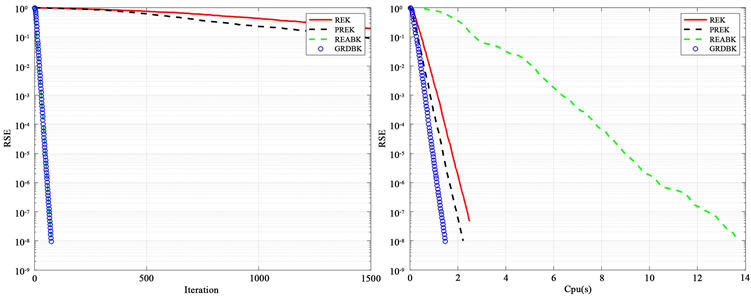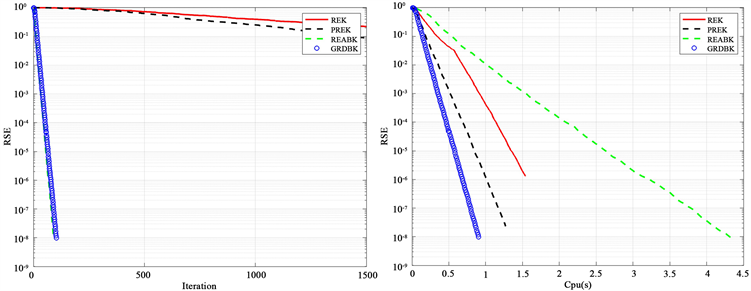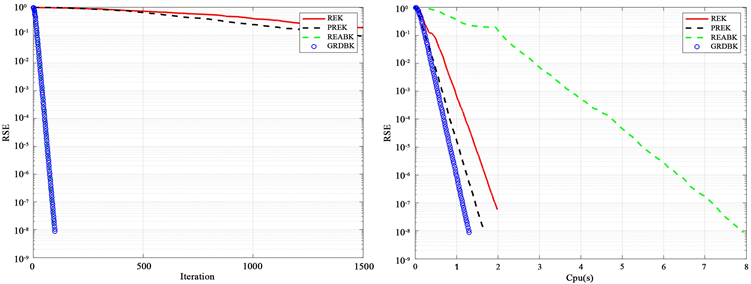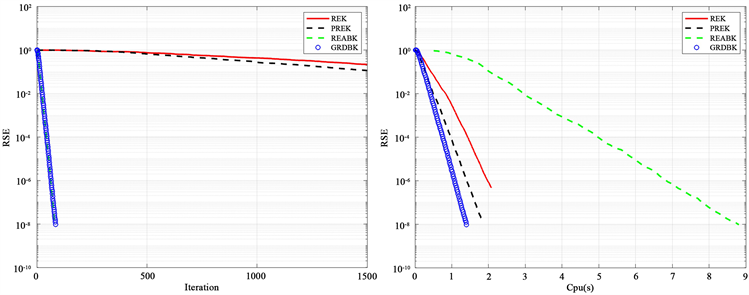#### 期刊菜单

Research on Greedy Random Extended Kaczmarz Algorithm
DOI: 10.12677/PM.2023.131013, PDF, HTML, XML, 下载: 42  浏览: 120

Abstract: The problem of solving large-scale linear systems of equations widely exists in various research fields. There are many methods to solve this problem, among which Kaczmarz iterative algorithm is more widely studied, and it is used for overdetermined and underdetermined systems. In this paper, we analyze the algorithm combining Kaczmarz extension algorithm with greedy algorithm at home and abroad, and propose greedy random double block Kaczmarz algorithm (GRDBK). GRDBK innovates on the basis of REK algorithm and GRK algorithm, and is suitable for overdetermined and inconsistent linear system.

1. 研究背景

$Ax=b$

${x}_{k+1}:={x}_{k}+{\alpha }_{k}\frac{{b}_{i}-〈{A}^{\left(i\right)},{x}_{k}〉}{{‖{A}^{\left(i\right)}‖}_{2}^{2}}{A}^{\left( i \right)}$

${x}_{k+1}:={x}_{k}+{\alpha }_{k}\frac{{b}_{r\left(i\right)}-〈{A}_{r\left(i\right)},{x}_{k}〉}{{‖{A}_{r\left(i\right)}‖}_{2}^{2}}{A}_{r\left( i \right)}$

2018年Bai和Wu  提出将贪婪算法与Kaczmarz相结合，得到贪心随机Kaczmarz (GRK)算法，该算法适用于求解大规模相容稀疏线性方程组，且近似解收敛到最小范数。GRK在RK的基础上，采用贪婪方法选择工作行。贪婪算法的主要思想是过滤掉残差向量r中较大的分量，最后剩下残差小的那些分量。在这些选定的行向量中，将残差向量r的范数之比作为选行概率，如下：

$\frac{{‖{\stackrel{^}{r}}_{k}\left({i}_{k}\right)‖}^{2}}{{‖{\stackrel{^}{r}}_{k}‖}_{2}^{2}}$

2013年Zouzias和Freris  在超定不相容的线性系统中，基于RK算法提出了扩展的随机Kaczmarz，分析了在不相容的情况下，方程 $Ax={b}_{R\left(A\right)}+r$ 中残差向量r的收敛情况，并给出了最小二乘解 ${x}_{k}$ 的收敛性定理。这个算法在讨论不相容线性系统的问题中，极具影响力，之后出现了很多基于REK的改进算法，例如2019年Bai和Wu  提出了部分随机扩展Kaczmarz (PREK)，2020年，Du和Si  提出了随机扩展双块Kaczmarz (REABK)。

2. 贪婪扩展随机块Kaczmarz (GRDBK)

2.1. GRDBK算法

2013年Zouzias和Freris  提出了REK算法，具体算法如下：

2018年，Bai和Wu发表了Kaczmarz贪婪算法，定义了根据残差向量选择活动行下标集的方法。具体算法如下：

2.2. 数值实例Figure 1. 9000 × 500Figure 2. 5000 × 500

3. 总结与展望Figure 3. 7000 × 500Figure 4. 8000 × 500

  Strohmer, T. and Vershynin, R. (2009) A Randomized Kaczmarz Algorithm with Exponential Convergence. Journal of Fourier Analysis & Applications, 15, Article No. 262. https://doi.org/10.1007/s00041-008-9030-4  Needell, D., Zhao, R. and Zouzias, A. (2015) Randomized Block Kaczmarz Method with Projection for Solving Least Squares. Linear Algebra & Its Applications, 484, 322-343. https://doi.org/10.1016/j.laa.2015.06.027  Zouzias, A. and Freris, N.M. (2013) Randomized Extended Kaczmarz for Solving Least Squares. SIAM Journal on Matrix Analysis and Applications, 34, 34773-34793. https://doi.org/10.1137/120889897  Bai, Z.Z. and Wu, W.T. (2018) On Greedy Randomized Kaczmarz Method for Solving Large Sparse Linear Systems. SIAM Journal on Scientific Computing, 40, A592-A606. https://doi.org/10.1137/17M1137747  Bai, Z.Z. and Wu, W.T. (2018) On Relaxed Greedy Randomized Kaczmarz Methods for Solving Large Sparse Linear Systems. Applied Mathematics Letters, 83, 21-26. https://doi.org/10.1016/j.aml.2018.03.008  Zhang, J.J. (2019) A new Greedy Kaczmarz Algorithm for the Solution of Very Large Linear Systems. Applied Mathematics Letters, 91, 207-212. https://doi.org/10.1016/j.aml.2018.12.022  Bai, Z.Z. and Wu, W.T. (2019) On Greedy Randomized Coordinate Descent Methods for Solving Large Linear Least-Squares Problems. Numerical Linear Algebra with Applications, 26, e2237. https://doi.org/10.1002/nla.2237  Zhang, J.H. and Guo, J.H. (2020) On Relaxed Greedy Randomized Coordinate Descent Methods for Solving Large Linear Least-Squares Problems. Applied Numerical Mathematics, 157, 372-384. https://doi.org/10.1016/j.apnum.2020.06.014  Niu, Y.Q. and Zheng, B. (2020) A Greedy Block Kaczmarz Algorithm for Solving Large-Scale Linear Systems. Applied Mathematics Letters, 104, Article ID: 106294. https://doi.org/10.1016/j.aml.2020.106294  杜亦疏, 殷俊锋, 张科. 求解大型稀疏线性方程组的贪婪距离随机Kaczmarz方法[J]. 同济大学学报(自然科学版), 2020, 48(8): 1224-1231. https://doi.org/10.11908/j.issn.0253-374x.20041  Liu, Y. and Gu, C.Q. (2021) On Greedy Randomized Block Kaczmarz Method for Consistent Linear Systems. Linear Algebra and Its Applications, 616, 178-200. https://doi.org/10.1016/j.laa.2021.01.024  Bai, Z.Z. and Wu, W.T. (2019) On Partially Randomized Extended Kaczmarz Method for Solving Large Sparse Overdetermined Inconsistent Linear Systems. Linear Algebra and Its Ap-plications, 578, 225-250. https://doi.org/10.1016/j.laa.2019.05.005  Du, K., Si, W.T and Sun, X.H. (2020) Randomized Extended Block Kaczmarz for Solving Least Squares. SIAM Journal on Scientific Computing, 42, A3541-A3559. https://doi.org/10.1137/20M1312629